407 493 6601

# High School Geometry - Equation of a Line

## Introduction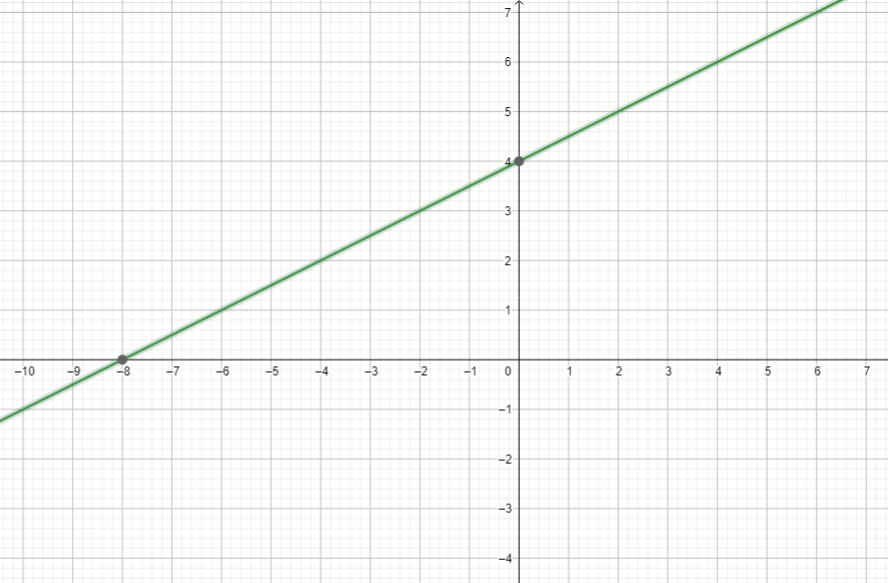• In this linear function graph, what do you think is the equation of the line?
• How did you come up with the equation of the line? Relate your steps.
• Basically, graphing linear functions have several methods to follow. This is the case when the equation of the line is given.
• To find the equation of a line when the graph is given or when points and slope are given, several methods must be followed.
• Recall the two cases of linear function below.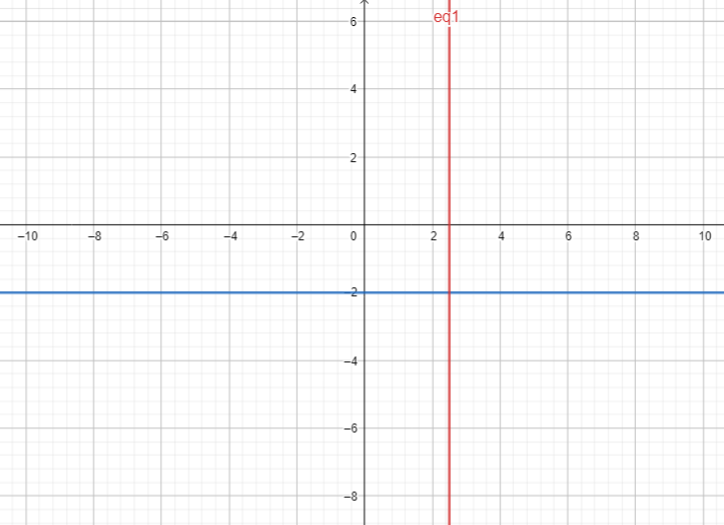• We can easily derive the equation of the horizontal line since it only involves a real constant value of y. The equation is simply $y=-2$ which has a slope of 0.
• It is also easy to write the equation of a vertical line because this involves a single value of x. The equation of the vertical line above is $x=\frac{5}{2}$ which has an undefined slope.
• What if the case involves finding the equation of an upward-sloping straight line or a downward-sloping straight line?

## Deriving the Equation of the Line

• There are many special forms of the equation of a line. The equation of a line is a relation between two variables that is satisfied by the coordinates of every point in the line.
• The following cases are as follows:

1. Slope and a point on the line are given. Use the point-slope form formula:

$y-{y}_{1}=m\left(x-{x}_{1}\right)$

2. Two Points on the Line are given. Use the two-point formula.

$y-{y}_{1}=\frac{{y}_{2}-{y}_{1}}{{x}_{2}-{x}_{1}}\left(x-{x}_{1}\right)$

3. Slope and y-intercept are given. Use the slope-intercept formula.

$y=mx+b$

4. X-intercept and y-intercept are given. Use the intercept formula.

## Solved Examples

Example 1. Find the equation of the line given the point $\left(5,6\right)$ and slope of $\frac{4}{5}$.

Solution:

Use the point-slope form formula.

$y-{y}_{1}=m\left(x-{x}_{1}\right)$

$y-6=\frac{4}{5}\left(x-5\right)$

$5y-30=4x-20$

Example 2. Find the equation of the line given the two points .

Solution:

Use the formula $y-{y}_{1}=\frac{{y}_{2}-{y}_{1}}{{x}_{2}-{x}_{1}}\left(x-{x}_{1}\right)$

By substitution of the values, we have: $y-4=\frac{-5-4}{-4-3}\left(x-3\right)$

Simplifying the equation yields

Example 3. What is the general equation of the line with a slope of 5 and y-intercept of 8?

Solution:

When slope and y-intercept are given, we use $y=mx+b$.

The equation of the line in slope intercept form is $y=5x+8$.

The general equation of this line is $5x-y+8=0$

Example 4. Find the equation of the line using the given points: x-intercept of 2 and y-intercept of 3.

Solution:

In this case, we use $\frac{x}{a}+\frac{y}{b}=1$

By substitution, we have:$\frac{x}{2}+\frac{y}{3}=1$.

In standard form, this is expressed as $3x+2y=6$.

## Parallel Lines and Perpendicular Lines

• If two or more lines are parallel, then their slopes are equal.
• If two lines are perpendicular, then the slope of one line is the negative reciprocal of the slope of the other line.

Example 1. What is the equation of the line that passes through $\left(3,-1\right)$ and is parallel to $y=3x-2$?

Solution:

If this line is parallel to $y=3x-2$, then its slope is 3.

Use the point-slope form equation $y-{y}_{1}=m\left(x-{x}_{1}\right)$.

$y+1=3\left(x-3\right)$

$y+1=3x-9$

$3x-y=10$

To check, the graph of  is shown below.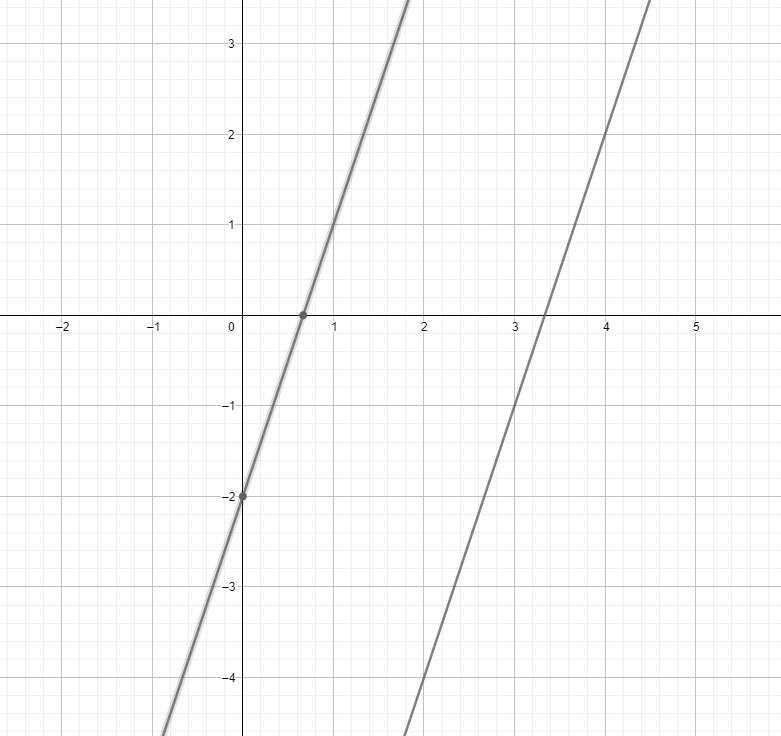Example 2. What is the equation of the line that passes through $\left(3,4\right)$ and is perpendicular to the line $y-4x-5=0$?

Solution:

The line $y-4x-4=0$ has a slope of 4. Therefore, the slope of the other line passing through $\left(3,4\right)$ is $-\frac{1}{4}$.

Using the point-slope form, we have:

$y-{y}_{1}=m\left(x-{x}_{1}\right)$

$y-4=-\frac{1}{4}\left(x-3\right)$

The equation of the line is

The graph of  is presented below: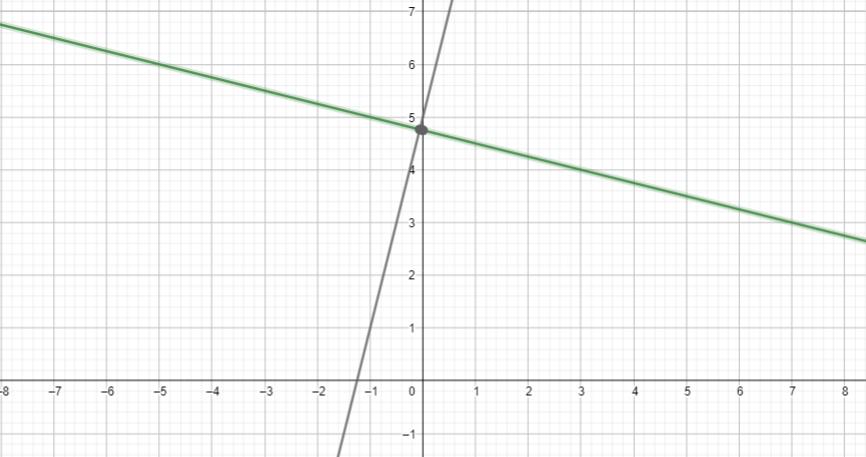Example 3. What is the equation of the line represented by its graph below?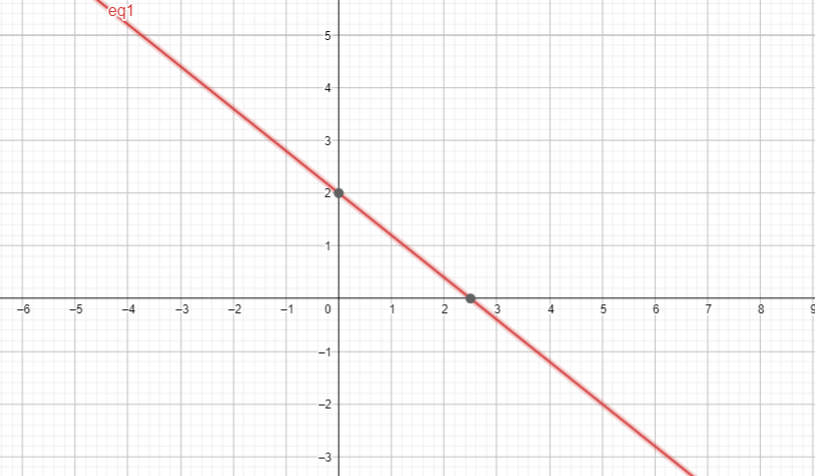Solution:

There are several ways to find the equation of this line when the graph is given. We can use any of the formulas discussed in the previous examples.

Using the Intercept formula, we identify the x-intercept and y-intercept.

$\frac{x}{a}+\frac{y}{b}=1$

## Cheat Sheet

• Point-Slope form Formula: $y-{y}_{1}=m\left(x-{x}_{1}\right)$
• Two-Point Formula: $y-{y}_{1}=\frac{{y}_{2}-{y}_{1}}{{x}_{2}-{x}_{1}}\left(x-{x}_{1}\right)$
• Slope Intercept Formula: $y=mx+b$
• Intercept Formula: $\frac{x}{a}+\frac{y}{b}=1$
• The equation of the line written in $ax+by=c$ is in a standard form or implicit form.
• The equation of the line expressed as $ax+by+c=0$ is in a general form.
• The slope of the horizontal straight line is 0.
• The slope of the vertical straight line is undefined.

## Blunder Areas

• A linear function can have only one x-intercept and one y-intercept.
• The graph of an equation in the form of $x=c$ is a vertical line through $\left(c,0\right)$. This does not represent a function.